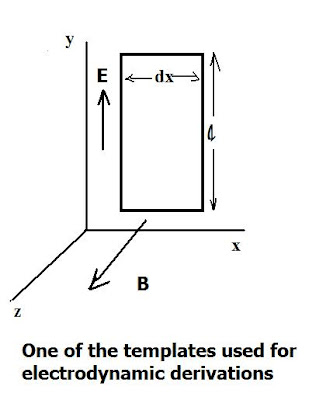## Tuesday, March 3, 2015

### Introducing Electrodynamics (Part 1)The physics discipline of electrodynamics plays a crucial role in both space physics and solar physics; From the aurora, to the magnetosphere's response to the solar wind and the triggering phase of certain solar flares, electrodynamics factors in. So, I introduce this three-part series of posts which surveys some of the fundamentals and also how they are applied to the solar physics setting.

let's begin by looking at some basic field derivations using the diagram shown, based on a rectangular wire loop placed in a crossed (E, B) field in a rectangular coordinate system (x,y, z). The field intensities E, B are shown in the directions indicated.

We begin by the general definition for the magnetic flux, φ:

φ = B (A)

where B is the magnetic induction and A the area of the region through which the flux passes.

Now, from the diagram, since the area of the wire loop is: A =  dx

Then the magnetic flux through it is:

φ= B dx

and the rate of change of magnetic flux (from Faraday's law) is:

dφ/dt = d/dt {B dx} = dB/dt (dx)

Further, taking the integral around the loops we find:

E d s  = -dφ/dt

->

E / x) dx  = - dx (B / t)

Thence: E / x    =   - B / t

Similarly,

B d s = mε  dx (d FE / d t  )

And the electric flux through the rectangle is expressed:

FE/ t dx  ( E / t)

where mo  is the magnetic permeability = 4 π x 10-7  H/m

and εis the electric permittivity of free space, viz.

εo = 8.85 x 10-12  F/m

- (¶B / x)   dx  =  mε  dx  ( E / t)

or finally,

(¶B / x)   =  - mε  ( E / t)

and if one takes the derivative of: E / x    =   - B / t

and combines with the preceding equation, one obtains the Maxwell wave equations:

1) 2E / x2  =   moεo  2 E / t2

2) ¶ 2B  / x2  =   moεo  2 B / t2

Note that in all these cases so far we are looking at plane wave solutions of the forms:

E= E(max) cos (kx - wt)

B = B(max) cos (kx - wt)

where the 'max' indices refer to maximum amplitude values of the field components, E, B, and w = 2 πf, where f is the frequency. We also may use the magnetic intensity H in the wave equations, which is related to B by:

B =  mo H

Using the above, interested readers ought to be able to show that the respective equations for E, B satisfy the Maxwell wave equations given!

Problems:

1) The generic wave equation for propagation of transverse waves, say on a string, is:

2y/ x2  =   1/ v2  2y/ t2

Where v is the wave velocity. For the Maxwell wave equations, however, we have v = c. Use this information to obtain the exact value of c - the speed of light - from one or other of the wave equations.

2) For a particular electromagnetic wave traveling in free space the power is given as P = 1300 Wm-2 .  Find the magnitudes of the wave vector components,  E y  and H z   if:

P = E y  H z / c

Hint: The impedance of free space is given by:

Ömo  / Ö εo    = E y  / H z

b)Find the magnitude of the magnetic flux density:  B z

3) Write out all the component wave equations for E.